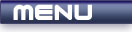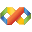Home Latest News Archive Tutorials C++ 2 3 4 Win32 Java OpenGL GLUT OpenGL ES 2 3 4 DirectX VB.NET HTML Photoshop 3dsmax Forums Projects Modelling Links About Us Contact Search Recent Tutorials C / C++ Win32 OpenGL OpenGL ES GLUT## Arrays

Tutorials > C++ > Arrays

### What are Arrays?

An array is essentially a variable that can be used to store multiple variables of the same data type.

### How are Arrays created?

Arrays are created using the form :

<data type> <var name>[<number of elements>];

eg. int ints will create an array of 5 integers.
You can also specify a start value as follows :

int nums[] = {3, 4, 5};

This will create an array of 3 integers with the values 3, 4 and 5 respectively.

### Why use Arrays?

You may have a set of data which can be logically grouped together eg. if you had a set of values for the amount of money you have received each month for the past year, instead of creating a new variable for each month eg.

int jan = 454;
int feb = 323;
.
.

you could have an array eg.

Contents of main.cpp :

```#include <iostream>
#include <stdlib.h>

using namespace std;

int main()
{
```

The following code creates a character array also known as a string. This string can consist of a maximum of 50 characters. An array of 10 integers is also created. Instead of creating a character array as follows :

char str = {'H', 'e', 'l', 'l', 'o' };

we can say

char str = "Hello";

This is obviously a much cleaner and simpler way of accomplishing things.

```	// Variable Declarations
//-----------------------------
char str = "Hello";
int nums;
```

Individual items of an array can be edited or assigned the same way as a normal variable by placing a [index] after the array name.

```	nums = 5;
nums = 1;
```

A character string can be printed out by using the printf function or cout object. How does the computer know to print only the string Hello and not all 50 characters.
The string is actually formed as follows :

'H' 'e' 'l' 'l' 'o' '\0'

The '\0' character is the NULL character as was explained along with escape characters. C / C++ knows to stop printing once a NULL character is found.

```	cout << str << endl;
```

As was shown above when assigning values to elements in an array, you are able to use the variable[index] the same way as a normal variable when performing operations on it eg. when outputting an element.

```	// Character Manipulation
//------------------------------
str = 'W';

cout << str << endl;
cout << str << endl;

cout << nums << endl;
```

The sizeof function can be used on an array to determine the size taken up in bytes. When outputting the size of the nums array we get 40 bytes as it consists of 10 integers where each integer takes up 4 bytes.
If we calculate the size of nums divided by the size of one integer, we will find out how many elements exist within the array.

```	// Array Capacity
//--------------------
cout << "Size of array in bytes : "
<< sizeof(nums) << " bytes" << endl;

cout << "Number of elements in array : "
<< (sizeof(nums) / sizeof(int)) << endl;
```

The last example shows how the size of an array can automatically be determined. You may leave out the value between [] as long as something is being assigned to it. In this case, the string "the" is being assigned. When checking the size of this array, you will notice that the size is 4 and not 3. This is because of the NULL character at the end of the string.

```	char the[] = "the";
cout << "Size of 'the' = " << sizeof(the) << endl;

system("pause");

return 0;
```

Ok. You should now have a fair understanding of the basics of arrays and what exactly an array is.

Please let me know of any comments you may have : Contact Me

 Source Files :< Tutorial 06 - Advanced Output Tutorial 08 - Pointers >